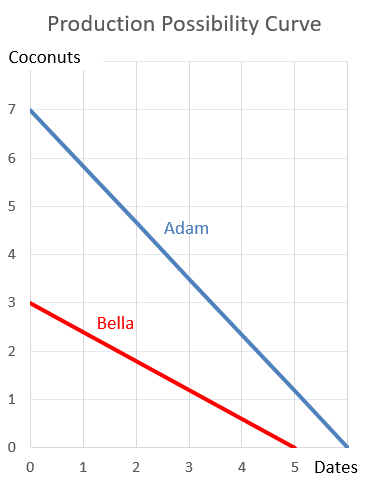# Fight Finance

#### CoursesTagsRandomAllRecentScores

An 'interest rate' is the same thing as a 'yield'. or ?

A three year corporate bond yields 12% pa with a coupon rate of 10% pa, paid semi-annually.

Find the effective six month yield, effective annual yield and the effective daily yield. Assume that each month has 30 days and that there are 360 days in a year.

All answers are given in the same order:

$r_\text{eff semi-annual}$, $r_\text{eff yearly}$, $r_\text{eff daily}$.

A share was bought for $10 (at t=0) and paid its annual dividend of$0.50 one year later (at t=1). Just after the dividend was paid, the share price was $11 (at t=1). What was the total return, capital return and income return? Calculate your answers as effective annual rates. The choices are given in the same order: $r_\text{total}$, $r_\text{capital}$, $r_\text{dividend}$. Your poor friend asks to borrow some money from you. He would like$1,000 now (t=0) and every year for the next 5 years, so there will be 6 payments of $1,000 from t=0 to t=5 inclusive. In return he will pay you$10,000 in seven years from now (t=7).

What is the net present value (NPV) of lending to your friend?

Assume that your friend will definitely pay you back so the loan is risk-free, and that the yield on risk-free government debt is 10% pa, given as an effective annual rate.

A moped is a bicycle with pedals and a little motor that can be switched on to assist the rider. Mopeds offer the rider:

The below screenshot of Microsoft's (MSFT) details were taken from the Google Finance website on 28 Nov 2014. Some information has been deliberately blanked out.What was MSFT's market capitalisation of equity?

For an asset price to triple every 5 years, what must be the expected future capital return, given as an effective annual rate?

Which Australian institution is in charge of monetary policy?

Adam and Bella are the only people on a remote island. Their production possibility curves are shown in the graph.

Assume that Adam and Bella cooperate according to the principle of comparative advantage.Which of the following statements is NOT correct?

The market's expected total return is 10% pa and the risk free rate is 5% pa, both given as effective annual rates.

A stock has a beta of 0.7.

In the last 5 minutes, bad economic news was released showing a higher chance of recession. Over this time the share market fell by 2%. The risk free rate was unchanged. What do you think was the stock's historical return over the last 5 minutes, given as an effective 5 minute rate?